## 2.12.1 Postulates of a Poisson Process

There are four postulates associated with the Poisson process: First we state them informally, then mathematically:

 1 The probability that at least one Poisson arrival occurs in a small time periodt is "approximately"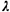t. Hereis called the arrival-rate parameter of the process. In applications, a numerical value foris found by measurement. Examples might be= 10 fire alarms per hour, or= 62 cars per hour passing through a tunnel, or= 8.3 unscheduled requests per day for a particular social service. 2 The number of Poisson-type arrivals happening in any prespecified time interval of fixed length is not dependent on the "starting time" of the interval or on the total number of Poisson arrivals recorded prior to the interval. For instance, if water-main breakdowns occur as a Poisson process, the number of breakdowns occurring in a particular day does not depend on the day being the tenth day of the month versus, say, the twentieth day of the month; nor does it depend on the number of breakdowns that occurred on the previous day or in the previous week. 3 The numbers of arrivals happening in disjoint time intervals are mutually independent random variables. Referring again to watermain breakdowns, say that we were interested in the number of breakdowns on September 28; this assumption would imply that knowledge (even partial knowledge) of the numbers of breakdowns on any days or combination of days other than September 28 would tell us nothing about the number of breakdowns on the 28th. 4 Given that one Poisson arrival occurs at a particular time, the conditional probability that another occurs at exactly the same time is zero. Thus, two or more arrivals cannot occur simultaneously. This may or may not be a good model for water-main breakdowns, but it certainly is not valid for the number of persons injured in auto accidents; given that an auto accident occurs at a particular time, it is an unfortunate fact that two or more persons may be injured at once.

These same four postulates can be stated mathematically more precisely:

 1 The probability that at least one Poisson arrival occurs in a time period of durationis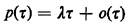where o() is a generic expression for a term or collection of terms that "goes to zero faster than kasgoes to zero" (for any constant k). Mathematically,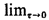o()/= 0. Note that for any finite sum of terms oN() = o1() + o2() + . . . + oN() such that oj()/0 as0 (j = 1, 2, . . ., N), we haveoN()= 0. 2 Let N(t) = total number of Poisson arrivals occurring in the interval [0, t]. We assume that N(0) = 0. For the interval {t1, t2}, the number of Poisson-type arrivals [N(t2) - N(t1)] (t2 > t1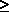0) is dependent only on (t2 - t1) and not on t1 or N(t1). 3 If 0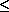t1 < t2t3 < t4. . ., the numbers of arrivals occurring in disjoint time intervals [N(t2) - N(t1)], [N(t4) - N(t3)], . . . are mutually independent random variables. 4 The probability that two or more Poisson arrivals occur in a time interval of lengthis o().
Given postulates 1-4, we now wish to prove the fundamental result for a Poisson process: that the number of Poisson arrivals occurring in a time interval of length t is Poisson-distributed with meant: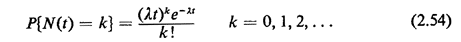Armed with this result, we can derive all the other interesting properties of a Poisson process.

Proof of (2.54): Let Pm(t) denote the probability that exactly m arrivals occur in time t,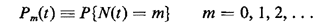We consider the time interval [0, t] and expand it by an amountas shown in Figure 2.12. We are interested in the probability that exactly m + 1 Poisson arrivals have occurred by time t +As shown in Figure 2.12, this event could occur with m + 1 arrivals occurring in [0, t] and no arrivals in [t, t +], or m arrivals in [0, t] and 1 arrival in [t, t +], and so on. Invoking independence of nonoverlapping intervals (postulate 3) and depen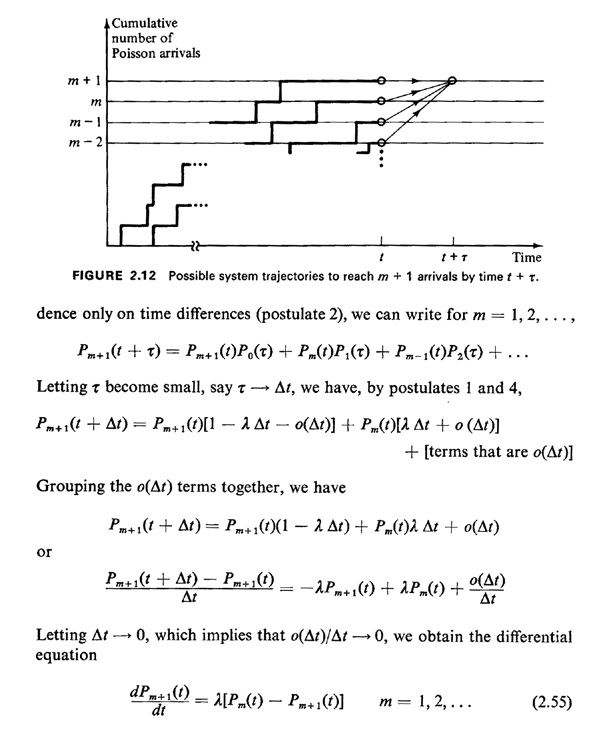This equation makes sense intuitively: it states that the time rate of change of the probability of exactly m + 1 arrivals by time t is equal to the probability of exactly m arrivals in time t multiplied by the rate at which a "transition occurs" from m to m + 1 arrivals, minus the probability of already having m + 1 arrivals by t multiplied by the rate at which a transition occurs from m + 1 to m + 2 arrivals; the conditional transition rate in each case is. Similar logic can be used to develop sets of coupled differential equations for more complicated processes, say whereis dependent on the number of previous arrivals or perhaps where arrivals can "depart," as in queueing systems. These ideas are expanded further in the discussion of "birth" processes in Chapter 3 and "birth-and-death" processes in Chapter 4.

While (2.55) holds for m = 1, 2, . . ., we also require an equation for m = 0. In a manner similar to the derivation above, we obtain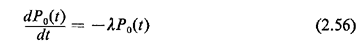The intuitive interpretation here is directly analogous to that of (2.55). The solution to (2.56) is clearly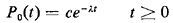with the constant c determined by the initial condition that P0(0) = 1, implying that c = 1. Then, by substitution into (2.55), one proves by induction that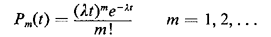This completes the proof.